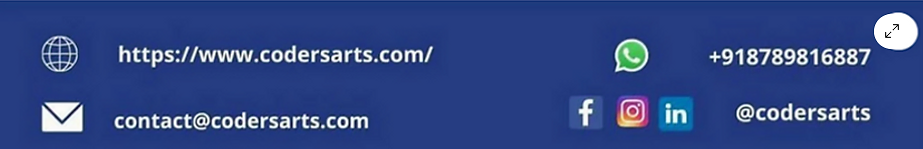top of page
Search

# Beginners' Guide to Classification using Iris Dataset

INTRODUCTION

To understand the world better, we need to comprehend each entity present in it. As a human, our experience leads us to make a final decision. But what if we are asked to identify a particular entity from millions of data and that too with a limited time and good accuracy? And what if we have to do this many times?

And this is where we use classification techniques of machine learning. Classification techniques help us to create machine learning models that identify things with great precision. One such identification problem is identifying variants of iris flower from the iris dataset. The Iris dataset consists of the information about three variants of iris flower, and we want to identify each variant with good accuracy.

While learning this, you will be introduced to various machine learning tasks such as encoding the string variables into numeric form as the machine learning models require the data to be in numeric form, and you will be introduced to feature scaling so that outliers won’t affect the performance of the model.

IMPORTING THE ESSENTIAL LIBRARIES

```# import the essential libraries

# to work the dataframe
import pandas as pd

# for visualizations
import matplotlib.pyplot as plt
import seaborn as sns

# scaling the features in order to reduce the effect the outliers
from sklearn.preprocessing import StandardScaler

# Importing the machine learning model
from sklearn.ensemble import RandomForestClassifier

# spliting the dataframe into train and test
from sklearn.model_selection import train_test_split

# for confusion matrix and classification report in order to evaluate the model
from sklearn.metrics import confusion_matrix
from sklearn.metrics import classification_report```

```# loading the datasetNow we will create a new data frame from the above data frame. This new data frame will have all the columns except for ID and Species. This is because ID is not a relevant feature to make the classification and Species is a target set.

```# removing the ID and label column from the dataset as
# ID is not a relevant feature
X = df.iloc[:, 1:-1]Now we want to see the values of target set.

```# getting the label column in order to train the model on labels
y = df.iloc[:, -1]
```0    Iris-setosa
1    Iris-setosa
2    Iris-setosa
3    Iris-setosa
4    Iris-setosa
Name: Species, dtype: object```

After this, we want to make sure that the dataset is not imbalance. To do this, we will use value_counts() method of Pandas data frame. If all the labels have equal value then the dataset is balanced otherwise it is imbalance.

```# checking if the labels are imbalance or not.
y.value_counts()```
```Iris-setosa        50
Iris-versicolor    50
Iris-virginica     50
Name: Species, dtype: int64```

After making sure that dataset is balanced, we want to convert the string labels into numerical form because machine learning model only understands numerical values. As there are only three unique values in the target set, we don't need to import any library for this. We can do this by using replace() method provided with the Pandas library. We will encode them in the form of label encoding.

```# performing label encoding
y = y.replace('Iris-setosa', 0)
y = y.replace('Iris-versicolor', 1)
y = y.replace('Iris-virginica', 2)
print(y.value_counts())```
```0    50
1    50
2    50
Name: Species, dtype: int64```

We will perform the standard scaling on the data frame to scale all the values close to zero. We do this to make sure that outliers won't affect that machine learning model while training.

```# performing standard scaling.
standard_scalar = StandardScaler()
standard_scalar.fit(X)
X_scaled = standard_scalar.transform(X)```
`print(X_scaled[:5])`
```[[-0.90068117  1.03205722 -1.3412724  -1.31297673]
[-1.14301691 -0.1249576  -1.3412724  -1.31297673]
[-1.38535265  0.33784833 -1.39813811 -1.31297673]
[-1.50652052  0.10644536 -1.2844067  -1.31297673]
[-1.02184904  1.26346019 -1.3412724  -1.31297673]]```

SPILITING THE DATA FRAME INTO TRAIN AND TEST

Splitting the data frame into train and test data frames. Train data frame consists 75 percent of the original data and test set consists 25 percent of the original dataset.

```# spliting the data into train and test
X_train, X_test, y_train, y_test = train_test_split(X_scaled, y, test_size = 0.25, random_state = 0, stratify = y)```

PERFORMING THE KNN CLASSIFIER

```from sklearn.neighbors import KNeighborsClassifier
knn_classifier = KNeighborsClassifier(n_neighbors = 5, metric = 'minkowski', p = 2)
knn_classifier.fit(X_train, y_train)```
`y_pred = classifier.predict(X_test)`

CLASSIFICATION REPORT FOR KNN CLASSIFIER

`print(classification_report(y_test, y_pred))`
```              precision    recall  f1-score   support

0       1.00      1.00      1.00        13
1       0.93      1.00      0.96        13
2       1.00      0.92      0.96        12

accuracy                           0.97        38
macro avg       0.98      0.97      0.97        38
weighted avg       0.98      0.97      0.97        38

```

According to the classification we have got the accuracy value 0.97. This means that we have archived the accuracy of 97 percent.

CONFUSION MATRIX FOR KNN CLASSIFIER

```knn_cm = confusion_matrix(y_test, y_pred)
cmap_value = 'CMRmap_r'
sns.heatmap(knn_cm, annot = True, cmap = cmap_value)
plt.show()```According to confusion matrix, one label is predicted as 1, but it should be predicted as 2.

Now we will perform Random-Forest classifier to see if it increases the model performance.

PERFORMING RANDOM FOREST CLASSIFICATION

```random_forest_classifier = RandomForestClassifier(n_estimators = 30, random_state = 42)
random_forest_classifier.fit(X_train, y_train)```
`y_pred = classifier.predict(X_test)`

CLASSIFICATION REPORT FOR RANDOM FOREST CLASSIFIER

`print(classification_report(y_test, y_pred))`
```              precision    recall  f1-score   support

0       1.00      1.00      1.00        13
1       1.00      1.00      1.00        13
2       1.00      1.00      1.00        12

accuracy                           1.00        38
macro avg       1.00      1.00      1.00        38
weighted avg       1.00      1.00      1.00        38```

As we can see, we have got the accuracy of 1.o. This means that we have archived the accuracy of 100 percent.

CONFUSION MATRIX FOR RANDOM FOREST CLASSIFIER

```random_forest_cm = confusion_matrix(y_test, y_pred)
cmap_value = 'CMRmap_r'
sns.heatmap(random_forest_cm, annot = True, cmap = cmap_value)
plt.show()```Finally, we have got all the prediction right according to confusion matrix.

If you are looking for help in Django project contact us contact@codersarts.combottom of page